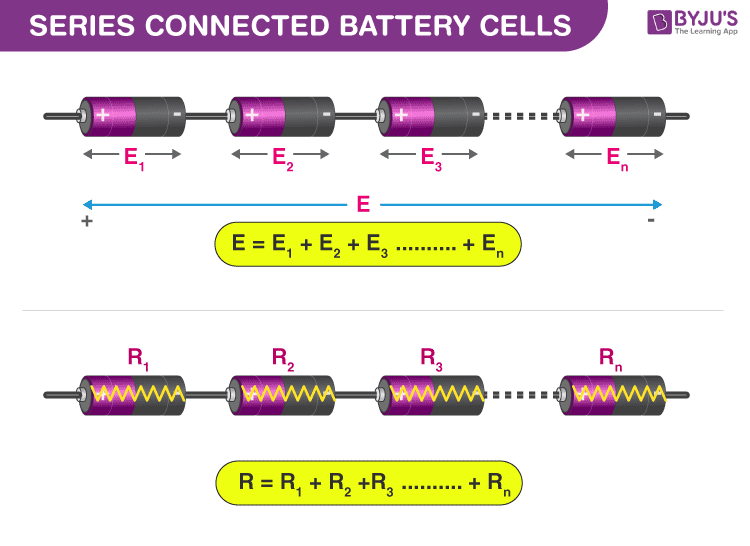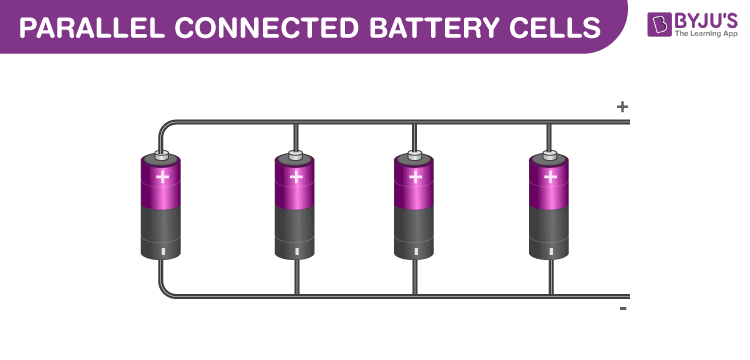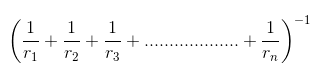# Batteries in Series and Parallel

The battery is a device that consists of one or more electrochemical cells with external connections for powering electrical appliances such as flashlights and mobile phones. Often, people mistakenly consider cell and battery to mean the same. But, it is essential to understand that a cell is a single unit that converts chemical energy into electrical energy, and a battery is a collection of cells.

The function of batteries and capacitors might seem similar as they both store and release electric energy. However, there are a few crucial differences between them that impact their potential applications due to which they function differently. Learn their differences from the link below:

## How does a battery work?

All batteries are made of three basic components an anode (the ‘-‘ side), a cathode (the ‘+’ side), and an electrolyte (a substance that chemically reacts with the anode and cathode). When an anode and a cathode is connected to a circuit, a chemical reaction takes place between the anode and the electrolyte. This reaction results in electrons flowing through the circuit and back into the cathode where another chemical reaction takes place. When the material in the cathode or anode is consumed or no longer able to be used in the reaction, the battery is unable to produce electricity.

### Important Terminologies to Know

#### EMF of a Battery

The voltage developed when no current flows through the battery is known as the emf of the battery. It is also referred to as the no-load voltage of the battery. Learn the difference between the emf of a battery and the voltage here.

#### Terminal Voltage of Battery

The terminal voltage of the battery is the voltage drawn from the battery when no current is being drawn. Because of the internal resistance of the battery, there will be a voltage drop across it. The terminal voltage of the battery is always lesser than the EMF of the battery.

#### Internal Resistance of the Battery

The resistance offered to the current by the battery as it flows across the negative terminal to the positive terminal is known as the internal resistance.

## Batteries in Series and Parallel Connections

There are two types of circuits: series and parallel. Batteries can either be connected in series, parallel or a combination of both. In a series circuit, electrons travel in one path and in the parallel circuit they travel through many branches. In this section, we will discuss in detail the series battery configuration and the parallel battery configuration.

### Series BatteriesA set of batteries are said to be connected in series when the positive terminal of one cell is connected to the negative terminal of the succeeding cell. The overall emf of the battery is the algebraic sum of all individual cells connected in series.

If E is the overall emf of the battery combined by n number of cells, then

E = E1 + E2 + E3 + E4 + ………..+ En

Similarly, if r1, r2, r3 are the internal resistances of individual cells, then the internal resistance of the battery will be equal to the sum of the internal resistance of the individual cells.

r = r1 + r2 + r3 + ……..+ rn.

### Parallel BatteriesA set of batteries are said to be connected in parallel when the positive terminals are connected together in a battery, and similarly, negative terminals of these cells are connected together. These combinations are referred to as parallel batteries. If emf of each cell is identical, then the emf of the battery combined by n numbers of cells connected in parallel is equal to the emf of each cell. The resultant internal resistance of the combination is,The current delivered by the battery is the sum of currents delivered by individual cells.

Learn various differences between series and parallel circuits from the link below:

### Mixed Grouping of Batteries

As discussed earlier, batteries can be connected in a mixture of both series and parallel. This combination is referred to as a series-parallel battery. Sometimes the load may require more voltage and current than what an individual battery cell can offer. For achieving the required load voltage, the desired numbers of battery are combined in series to achieve the required current and these series combinations are connected in parallel.

### Solved Problems

1) What is the internal resistance of a cell of emf 1.5 V that can deliver a maximum current of 3 A?

Solution:

For maximum current, the load resistance should be 0.

E = Ir

Rearranging the equation, we get

$E=\frac{I}{r}$

Substituting the values, we get

$E=\frac{1.5}{3}= 0.5 \Omega$

The internal resistance of the cell is 0.5 Ω.

2) List the factors affecting the internal resistance of the cell.

Solution:

The internal resistance of a cell depends on the following factors:

• The surface area of the electrodes
• The distance between the electrodes
• Nature and the concentration of the electrolyte
• The temperature of the electrolyte

Stay tuned to BYJU’S and fall in love with learning!